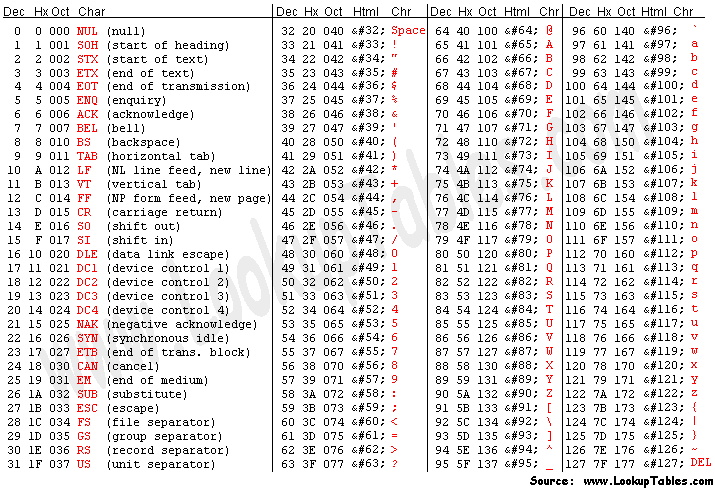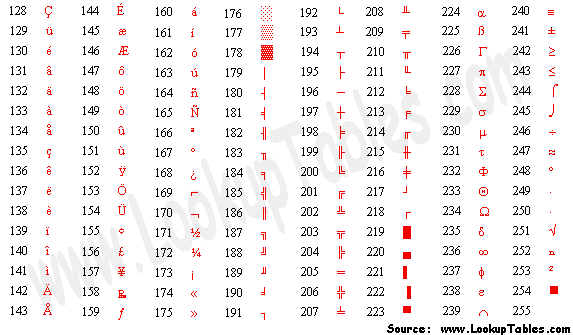# Lost Memory

Programming is an art, then be an artist.

C++博客 :: 首页 :: 联系 :: 聚合:: 管理

•

• 积分 - 11665
• 排名 - 1049

### 评论排行榜

2015年3月7日 #

摘要:   阅读全文
posted @ 2015-03-07 17:11 pamilty 阅读(2531) | 评论 (0)编辑 收藏

2014年1月20日 #

reference link --- [Comparison : http://floating-point-gui.de/errors/comparison/]

# Comparison

Due to rounding errors, most floating-point numbers end up being slightly imprecise. As long as this imprecision stays small, it can usually be ignored. However, it also means that numbers expected to be equal (e.g. when calculating the same result through different correct methods) often differ slightly, and a simple equality test fails. For example:

``	float a = 0.15 + 0.15 	float b = 0.1 + 0.2 	if(a == b) // can be false! 	if(a >= b) // can also be false! ``

## Don’t use absolute error margins

The solution is to check not whether the numbers are exactly the same, but whether their difference is very small. The error margin that the difference is compared to is often called epsilon. The most simple form:

``	if( Math.abs(a-b) < 0.00001) // wrong - don't do this ``

This is a bad way to do it because a fixed epsilon chosen because it “looks small” could actually be way too large when the numbers being compared are very small as well. The comparison would return “true” for numbers that are quite different. And when the numbers are very large, the epsilon could end up being smaller than the smallest rounding error, so that the comparison always returns “false”. Therefore, it is necessary to see whether the relative error is smaller than epsilon:

``	if( Math.abs((a-b)/b) < 0.00001 ) // still not right! ``

## Look out for edge cases

There are some important special cases where this will fail:

• When both `a` and `b` are zero. `0.0/0.0` is “not a number”, which causes an exception on some platforms, or returns false for all comparisons.
• When only `b` is zero, the division yields “infinity”, which may also cause an exception, or is greater than epsilon even when `a` is smaller.
• It returns `false` when both `a` and `b` are very small but on opposite sides of zero, even when they’re the smallest possible non-zero numbers.

Also, the result is not commutative (`nearlyEquals(a,b)` is not always the same as `nearlyEquals(b,a)`). To fix these problems, the code has to get a lot more complex, so we really need to put it into a function of its own:

``	public static boolean nearlyEqual(float a, float b, float epsilon) { 		final float absA = Math.abs(a); 		final float absB = Math.abs(b); 		final float diff = Math.abs(a - b);  		if (a == b) { // shortcut, handles infinities 			return true; 		} else if (a == 0 || b == 0 || diff < Float.MIN_NORMAL) { 			// a or b is zero or both are extremely close to it 			// relative error is less meaningful here 			return diff < (epsilon * Float.MIN_NORMAL); 		} else { // use relative error 			return diff / (absA + absB) < epsilon; 		} 	} ``

This method passes tests for many important special cases, but as you can see, it uses some quite non-obvious logic. In particular, it has to use a completely different definition of error margin when `a` or `b` is zero, because the classical definition of relative error becomes meaningless in those cases.

There are some cases where the method above still produces unexpected results (in particular, it’s much stricter when one value is nearly zero than when it is exactly zero), and some of the tests it was developed to pass probably specify behaviour that is not appropriate for some applications. Before using it, make sure it’s appropriate for your application!

## Comparing floating-point values as integers

There is an alternative to heaping conceptual complexity onto such an apparently simple task: instead of comparing `a`and `b` as real numbers, we can think about them as discrete steps and define the error margin as the maximum number of possible floating-point values between the two values.

This is conceptually very clear and easy and has the advantage of implicitly scaling the relative error margin with the magnitude of the values. Technically, it’s a bit more complex, but not as much as you might think, because IEEE 754 floats are designed to maintain their order when their bit patterns are interpreted as integers.

However, this method does require the programming language to support conversion between floating-point values and integer bit patterns. Read the Comparing floating-point numbers paper for more details.

posted @ 2014-01-20 11:47 pamilty 阅读(624) | 评论 (0)编辑 收藏

2013年7月15日 #

*
open Terminal and type the following command:

\$sudo sh -c 'echo "greeter-show-manual-login=true" >> /etc/lightdm/lightdm.conf'

restart computer and choose to login as the desired user.

reference link: Liberian Geek Login as Root in Ubuntu 12.04 (Precise Pangolin)
posted @ 2013-07-15 13:13 pamilty 阅读(329) | 评论 (0)编辑 收藏

1) First
enable the root account by running the commands below:
\$ sudo passwd -u root
2) Then
\$sudo passwd root
When you run the commands above, you'll get prompted to enter a new password.

reference link:  Liberian Geek [Question] What is the Root Default Password in Ubuntu 12.04?
posted @ 2013-07-15 11:40 pamilty 阅读(396) | 评论 (0)编辑 收藏

2013年5月2日 #

Precedence Operator Description Associativity
1

highest

`::` Scope resolution (C++ only) None
2 `++` Suffix increment Left-to-right
`--` Suffix decrement
`()` Function call
`[]` Array subscripting
`.` Element selection by reference
`->` Element selection through pointer
`typeid()` Run-time type information (C++ only) (see typeid)
`const_cast` Type cast (C++ only) (see const_cast)
`dynamic_cast` Type cast (C++ only) (see dynamic_cast)
`reinterpret_cast` Type cast (C++ only) (see reinterpret_cast)
`static_cast` Type cast (C++ only) (see static_cast)
3 `++` Prefix increment Right-to-left
`--` Prefix decrement
`+` Unary plus
`-` Unary minus
`!` Logical NOT
`~` Bitwise NOT (One's Complement)
`(type)` Type cast
`*` Indirection (dereference)
`&` Address-of
`sizeof` Size-of
`new`, `new[]` Dynamic memory allocation (C++ only)
`delete`, `delete[]` Dynamic memory deallocation (C++ only)
4 `.*` Pointer to member (C++ only) Left-to-right
`->*` Pointer to member (C++ only)
5 `*` Multiplication Left-to-right
`/` Division
`%` Modulo (remainder)
6 `+` Addition Left-to-right
`-` Subtraction
7 `<<` Bitwise left shift Left-to-right
`>>` Bitwise right shift
8 `<` Less than Left-to-right
`<=` Less than or equal to
`>` Greater than
`>=` Greater than or equal to
9 `==` Equal to Left-to-right
`!=` Not equal to
10 `&` Bitwise AND Left-to-right
11 `^` Bitwise XOR (exclusive or) Left-to-right
12 `|` Bitwise OR (inclusive or) Left-to-right
13 `&&` Logical AND Left-to-right
14 `||` Logical OR Left-to-right
15 `?:` Ternary conditional (see ?:) Right-to-left
16 `=` Direct assignment Right-to-left
`+=` Assignment by sum
`-=` Assignment by difference
`*=` Assignment by product
`/=` Assignment by quotient
`%=` Assignment by remainder
`<<=` Assignment by bitwise left shift
`>>=` Assignment by bitwise right shift
`&=` Assignment by bitwise AND
`^=` Assignment by bitwise XOR
`|=` Assignment by bitwise OR
17 `throw` Throw operator (exceptions throwing, C++ only) Right-to-left
18

lowest

`,` Comma Left-to-right

reference url: http://en.wikipedia.org/wiki/Operators_in_C_and_C%2B%2B
posted @ 2013-05-02 13:06 pamilty 阅读(441) | 评论 (0)编辑 收藏

2013年2月23日 #

Unicode 值对应的 UTF-8 编码查询地址：http://www.utf8-chartable.de/
ASCII 码表，及扩展表内容如下：Extended ASCII tableposted @ 2013-02-23 22:32 pamilty 阅读(7237) | 评论 (0)编辑 收藏